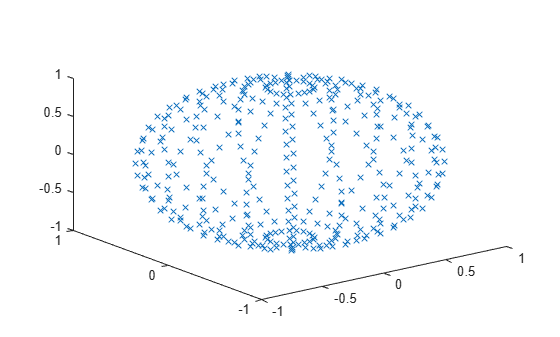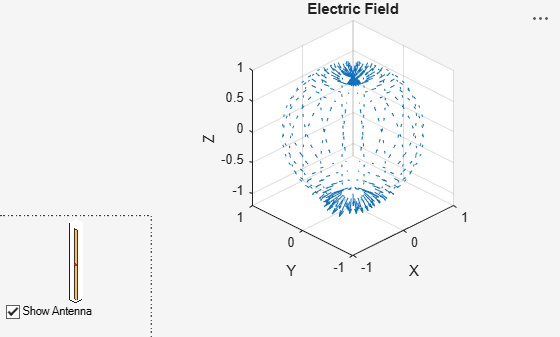## Antenna Near-Field Visualization

This example shows how to calculate and visualize the near-fields for antennas. Near fields can be plotted in Antenna Toolbox™ using the `EHfields` function. This function can also be used to calculate and plot the near-fields for antenna arrays.In the near field region, the electric and magnetic fields can exist independently of each other, and one type of field can dominate the other.

### Create Dipole Antenna

Create the dipole antenna in the default configuration.

```ant = dipole; show(ant);```### Define Points to Calculate Near-Field

The near fields as the name suggest are fields that are calculated in the close proximity of the antenna. In this example, we plot the fields over a sphere of diameter 2 meters and centered at the origin. The dipole is also of length two meters and centered at the origin. So the sphere completely encloses the dipole. The sphere command generates 21 x 21 matrices for X, Y and Z. They are combined to generate 441 points as shown below.

```[X, Y, Z] = sphere(20); Points = [X(:), Y(:), Z(:)].'; plot3(Points(1,:), Points(2,:), Points(3,:), 'x');```### Plot Near-Fields

The near-fields at the various points calculated above can be plotted by using the EHfields function as shown. By default both the electric and magnetic fields are plotted.

`EHfields(ant, 70e6, Points);`Individual fields can be plotted by using the `Viewfield` flag. Below we plot only the magnetic field. As expected, the direction of the magnetic field follows the right hand rule due to the current along the length of the dipole.

`EHfields(ant, 70e6, Points, 'ViewField', 'H');`### Plot Electric Field

The plot below shows the electric field distribution over the points. As expected, we have electric field lines coming out of the positive charge and into the negative charge. The electric field forms loops along the length of the dipole. The `ScaleFields` flag is used to scale the size of the field vector. In this case, the electric field vector is multiplied by 2, to artificially increase the size of the vectors so as to make them clearly visible.

`EHfields(ant, 70e6, Points, 'ViewField', 'E', 'ScaleFields', [2,0]);`### Output Near-Fields to Workspace

By providing RHS, the electric and magnetic fields can be outputted to the workspace to perform any other mathematical computations.

`[E, H] = EHfields(ant, 70e6, Points);`

Just to clarify, the EHfields function, calculates the E-H fields at any point in space. It can be used to calculate the near or the far fields.